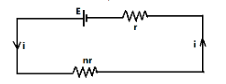QuestionAnswers

# A cell of E.M.F. $E$ and internal resistance $r$ is connected in series with an external resistance $nr$ then the ratio of the terminal potential difference to E.M.F. isA.)$\dfrac{1}{n}$B.)$\dfrac{1}{{n + 1}}$C.)$\dfrac{n}{{n + 1}}$D.)$\dfrac{{n + 1}}{n}$Verified
128.7k+ views
Hint: Here the given situation will give a simple circuit having a resistance $nr$ and a battery of emf $E$ and internal resistance $r$, and in a circuit we can apply the Kirchhoff’s voltage law to get the terminal potential difference across the given circuit.

We will draw a circuit diagram having battery of emf $E$ and internal resistance $r$ and an external resistance of $nr$, which is shown belowSo above is the required diagram as per the given condition in the questions.
Let the terminal potential difference of the circuit =$V$
So now applying the Kirchhoff’s voltage law in the above circuit, we get,
$V = E - ir$-------equation (1)
Also, we can get the value of the current flowing the circuit as follows,
$i = \dfrac{{E.M.F.}}{{total{\text{ }}resistance}}$
Also, we can calculate the total resistance, since internal resistance and the external resistance both are in the series combination, so the equivalent resistance will be,
${R_{eq}} = r + nr$
So now the current flowing in the circuit will be,
$i = \dfrac{E}{{r + nr}}$
So now from the equation (1)
$V = E - \left[ {\dfrac{E}{{r + nr}}} \right]r$
$\Rightarrow V = E - \left[ {\dfrac{E}{{(n + 1)r}}} \right]r$
$\Rightarrow V = E\left[ {1 - \dfrac{1}{{(n + 1)}}} \right]$
$\Rightarrow V = E\left[ {\dfrac{{n + 1 - 1}}{{(n + 1)}}} \right]$
$\Rightarrow V = E\left[ {\dfrac{n}{{(n + 1)}}} \right]$
$\Rightarrow V = \dfrac{{En}}{{(n + 1)}}$----equation (2)
Hence the terminal potential difference for the circuit is $\dfrac{{En}}{{(n + 1)}}$.
Now we have to calculate the ratio of the terminal potential difference to E.M.F., and we know the value of both terminal potential difference and the E.M.F.
So, the ratio of the terminal potential difference to E.M.F. =$\dfrac{V}{E}$
$= \dfrac{{\left[ {\dfrac{{En}}{{(n + 1)}}} \right]}}{E}$ (on putting the value of $V$from the equation (2))
$= \dfrac{n}{{(n + 1)}}$
So, the ratio of the terminal potential difference to E.M.F. is $\dfrac{n}{{n + 1}}$.

So, the correct answer is “Option C”.

Note:
In the above problem we have seen the mention of the internal resistance and the external resistance in a circuit. Internal resistance is always related to the battery or cell. Internal resistance is the resistance to the flow of the current by the electrolyte inside a battery, while the external resistance is the resistance to the flow of the current in the external circuit.